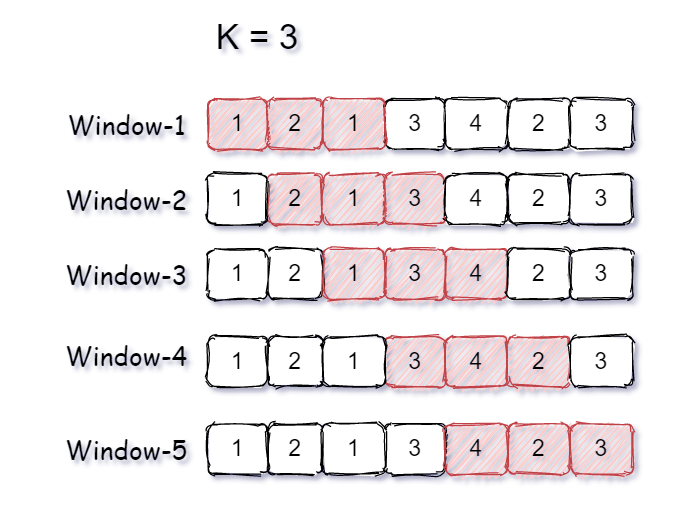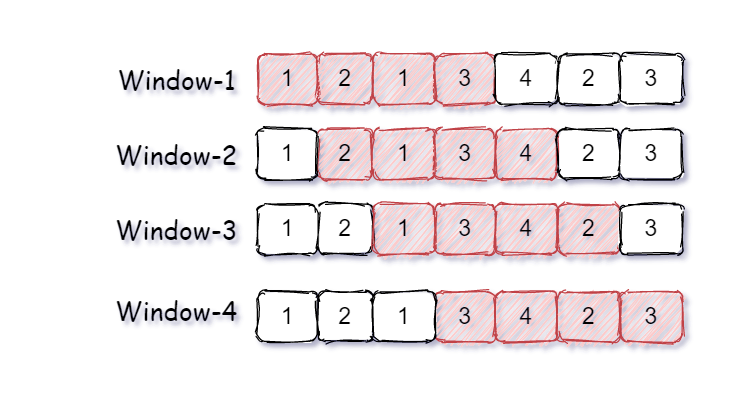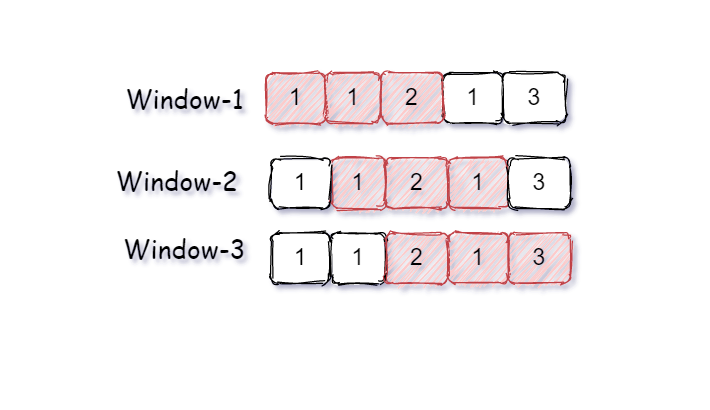New update is available. Click here to update.Close
Topic list
Count Distinct Element in Every K Size Window
EASY
15 mins35 upvotes
Arrays
Topics (Covered in this problem)
Problem solved
Skill meterArrays
-
-
Other topics
Problem solved
Skill meterStrings
-
-Matrices (2D Arrays)
-
--
-Sorting
-
-Binary Search
-
-Stacks & Queues
-
-Trees
-
-Graph
-
-Dynamic Programming
-
-Greedy
-
-Tries
-
-SQL
-
-Binary Search Trees
-
-Heap
-
-Bit Manipulation
-
-
Solve problems & track your progress
Checkout your overall progress in every topic here
BecomeSensei
in DSA topics
Open the topic and solve more problems associated with it to improve your skills
Check out the skill meter for every topic
See how many problems you are left with to solve for cracking any stage. Score more than zero to get your progress counted.

# Count Distinct Element in Every K Size Window

Easy0/40
Avg time to solve 15 mins
Success Rate 85 %Share35 upvotes

## Problem Statement

#### You are given an array ‘ARR’ of size ‘N’ and an integer ‘K’. Your task is to find the total number of distinct elements present in every ‘K’ sized window of the array. A ‘K’ sized window can also be viewed as a series of continuous ‘K’ elements present in the sequence.

##### Note:
``````1. The size of ‘ARR’ will always be greater than or equal to the ‘K’.
2. Here window refers to a subarray of ‘ARR’. Hence ‘K’ sized window means a subarray of size ‘K’.
3. You are not required to print the output explicitly. It has already been taken care of. Just implement the function and return an array of the count of all distinct elements in the ‘K’ size window.
``````

#### Example

``````Consider ARR = [ 1, 2, 1, 3, 4, 2, 3 ] and K = 3.
````````````As per the given input, we have a sequence of numbers of length 7, and we need to find the number of distinct elements present in all the windows of size 3.

Window-1 has three elements { 1, 2, 1 } and only two elements { 1, 2 } are distinct because 1 is repeating two times.
Window-2 has three elements { 2, 1, 3 } and all three elements are distinct { 2, 1, 3 }.
Window-3 has three elements { 1, 3, 4 } and all three elements are distinct { 1, 3, 4 }.
Window-4 has three elements { 3, 4, 2 } and all three elements are distinct { 3, 4, 2 }.
Window-5 has three elements { 4, 2, 3 } and all three elements are distinct { 4, 2, 3 }.

Hence, the count of distinct elements in all K sized windows is { 2, 3, 3, 3, 3 }.
``````
Detailed explanation ( Input/output format, Notes, Constraints, Images )##### Sample Input 1:
``````2
7 4
1 2 1 3 4 2 3
5 3
1 1 2 1 3
``````
##### Sample Output 1:
``````3 4 4 3
2 2 3
``````
##### Explanation of sample input 1:
``````Test Case 1:
````````````Window-1 has four elements { 1, 2, 1, 3 } and only three elements { 1, 2, 3 } are distinct because 1 is repeating two times.
Window-2 has four elements { 2, 1, 3, 4 } and all four elements { 2, 1, 3, 4 } are distinct.
Window-3 has four element { 1, 3, 4, 2 } and all four elements { 1, 3, 4, 2 } are distinct.
Window-4 has four element { 3, 4, 2, 3 } and only three elements { 3, 4, 2 } are distinct because 3 is repeating two times.

Hence, the count of distinct elements in all windows is { 3, 4, 4, 3}.

Test case 2:
````````````Window-1 has three elements { 1, 1, 2 } and only two elements { 1, 2 } are distinct because 1 is repeating two times.
Window-2 has three elements { 1, 2, 1 } and only two elements { 2, 1 } are distinct.
Window-3 has three elements { 2, 1, 3 } and all three elements { 2, 1, 3 } are distinct.

Hence, the count of distinct elements in all windows is { 2, 2, 3 }.
``````
##### Sample Input 2:
``````2
4 1
2 3 1 2
5 2
2 2 3 2 1
``````
##### Sample Output 2:
``````1 1 1 1
1 2 2 2
``````AutoConsole